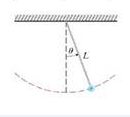Chapter 17.3, Problem 18E

Chapter
Section
Textbook Problem

The figure shows a pendulum with length I, and the angle θ from the vertical to the pendulum. It can be shown that θ, as a function of time, satisfies the nonlinear differential equation d 2 θ d t 2 + g L sin θ = 0 where g is the acceleration due to gravity. For small values of θ we can use the linear approximation sin θ = θ and then the differential equation becomes linear.(a) Find  the equation of motion of a pendulum with length 1 m. if θ is initially 0.2 rad and the initial angular velocity is d θ/dt =1 rad/s.(b) What is the maximum angle from the vertical?(c) What is the period of the pendulum (that is, the time to complete one back-and-forth swing)?(d) When will the pendulum first be vertical?(e) What is the angular velocity when the pendulum is vertical?(a)

To determine

To find: The equation of motion of a pendulum with length.

Explanation

Given data:

sinθθ , L=1m , θ(0)=0.2rad , dθ(0)dt=1rads , d2θdt2+gLsinθ=0 .

Formula used:

Write the expression for general solution complex roots.

θ(t)=eαt[c1cos(βt)+c2sin(βt)] (1)

Write the expression for r .

r=α+βi (2)

Write the expression for auxiliary equation.

ar2+br+c=0 (3)

Write the expression for differential equation.

ay+by+cy=0 (4)

Consider the expression for differential equation as follows.

d2θdt2+gLsinθ=0 (5)

Consider the value of g as follows.

g=9.8

Substitute 9.8 for g , 1 for L and θ for sinθ in equation (5),

d2θdt2+9.81θ=0

d2θdt2+9.8θ=0 (6)

Modify equation (6) as follows.

θ+9.8θ=0 (7)

Modify equation (4) as follows.

aθ+bθ+cθ=0 (8)

Compare equation (7) and (8).

a=1b=0c=9.8

Substitute 1 for a , 0 for b and 9.8 for c in equation (3),

(1)r2+(0)r+9.8=0r2+9.8=0r2=9.8

r=±9.8i (9)

Compare equation (2) and (9).

α=0β=9.8

Substitute 0 for α and 9.8 for β in equation (1),

θ(t)=e(0)t[c1cos(9

(b)

To determine

To find: The maximum angle from the vertical.

(c)

To determine

To find: The period of the pendulum.

(d)

To determine

To find: The time at which pendulum should be vertical for first time.

(e)

To determine

To find: The angular velocity when the pendulum is vertical.

Still sussing out bartleby?

Check out a sample textbook solution.

See a sample solution

The Solution to Your Study Problems

Bartleby provides explanations to thousands of textbook problems written by our experts, many with advanced degrees!

Get Started

Convert the expressions in Exercises 8596 radical form. 94(1x)7/3

Finite Mathematics and Applied Calculus (MindTap Course List)

For y = x sin x, y = _____. a) x cos x b) x cos x + 1 c) cos x d) x cos x + sin x

Study Guide for Stewart's Single Variable Calculus: Early Transcendentals, 8th

True or False: If F is conservative, then curl F = 0.

Study Guide for Stewart's Multivariable Calculus, 8th